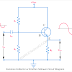## What is Emitter Follower Circuit?

The emitter follower circuit is basically a transistor amplifier circuit where the load is connected to the emitter terminal of the transistor. Here, the input signal is applied to the base terminal of the transistor, and the output is taken from the emitter terminal.

It is known as an Emitter Follower circuit because the output or emitter voltage exactly follows the changes in the base or input voltage. The name is derived from the term 'follows' or 'following'.

As the emitter voltage deeply follows the changes in the Base voltage, the changes in the emitter and base voltage happen exactly the same. That means in this circuit the voltage gain is unity and the both input and output voltages are in the same phase. The output voltage doesn't shift by 180 degrees angle.

The emitter follower circuit is also known as a common collector(CC) circuit. Because here the load is connected or output is taken from the emitter terminals instead of the collector terminal. And the collector terminal is effectively grounded or earthed through the power supply.

The emitter follower or common collector circuit is just opposite to the common emitter(CE) circuit. In the CE configuration, the output is taken from the collector terminal and the emitter terminal is grounded but here in the CC configuration the output is taken from the emitter terminal, and the collector is connected to the ground.

## Emitter Follower Circuit Diagram

Here, you can see a basic circuit diagram of emitter follower circuit or common collector circuit.

Here, an NPN transistor is used to build this circuit. A voltage divider circuit is used to bias the transistor to bring it into the conduction stage. The resistors R1 and R2 form the voltage divider network. It is a very straightforward circuit. Also, you can see the circuit is built in such a way that the forward junction will be formed between the base and emitter terminal. So the input signal is applied to the base can easily passed to the emitter through the forward junction therefore the output signal will be in the same phase as the input signal.

## Emitter Follower Circuit Properties or Characteristics

The common characteristics of emitter follower or common collector circuit are,
1. High Input Resistance or Impedance
2. Low Output Resistance Impedance
3. Zero Collector Resistance or Impedance
4. Low input current circulation
5. High Output Current Circulation
6. Voltage approximately Unity.
7. Input and output are in the same phase.

The emitter follower circuit is also a negative current feedback circuit because when the input voltage Vs is applied to the base terminal, the emitter terminal circulates an electric current Ie. This emitter current Ie develops an Output Voltage across the Emitter Resistor RE that is equal to the IeRe. This voltage will oppose the AC signal voltage Vs as it is in phase opposition to Vs. The output voltage feedback to the input is proportional to the emitter current.

Also note that, as the positive and negative half cycles occur in the input and output signal at the same time interval, the output signal is always in the same phase as the input signal.

## Application and Uses of Emitter Follower Circuit

1. They are used for Impedance Circuit
2. It can be used as a voltage buffer circuit.
3. They are used for voltage-controlled current source applications.
4. It is used as a voltage-sensing circuit.
5. It is used in signal generator circuits.

What is Emitter Follower Circuit? Diagram, Properties, UsesReviewed by Author on May 11, 2023 Rating: 5